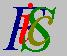Mauro Murzi's pages on Philosophy of Science - Quantum mechanics
Complex functions of real variablesExamples of geometrical representation Derivative# [2. Mathematical elements.]

## Complex functions of real variables.

Let X and Y be two sets and let be a rule that assigns to every element of X exactly one element of Y; is called a function from X to Y and the sets X and Y are called respectively the domain and the co-domain (or range) of the function f. A function from X to Y is represented by the notation y = f (x) where y is the element of Y assigned to the element x of X. The element x is called the argument of the function and the element y is called the value of for the argument x. The elements x and y are also called variables; x is the independent variable and y is the dependent variable.

If the domain and the co-domain of a function are equal to the set R of real numbers (that is: X=Y=R) then the function is called a real function of a real variable. A well known function of this kind is the function square ( y = x² ) which associates to every real number x its square x².

Multiplication between two real numbers is a real function of two real variables; it is an example of functions of the type y = f (x1 , x2) where x1 and x2 are real numbers. From a formal point of view, a function like y = f (x1 , x2) with x1 and x2 real numbers is a function from RxR to R, where RxR is the Cartesian product of R by itself: The domain of is the set of all ordered pairs of real numbers, that is the set whose elements are the ordered pairs < ab > for every real number a and b.

Complex functions of a real variable are functions from the set R of real number to the set C of complex numbers; a function of this kind associates to every real number exactly one complex number. An example is the square root function that assign to every real number its square root: If the argument of this function is less than zero, then the value of the function is a complex number.

A complex function of n real variables is a function whose arguments are n real numbers and whose value is a complex number. It is a function from Rn to C.

From this point, Greek letters will be used to denote complex functions or complex numbers; Latin letters will be used to denote real functions or real numbers. Here are some examples:

• φ (x) and Ψ (x) denote complex functions of a real variable.
• φ (xy) and Ψ (xyz) denote complex functions of real variables (two and three real variables, respectively).
• φ  and Ψ  denote complex functions of a real variables, without specifying the number of real variables.
• σ  denotes a complex number.
• x y z denote real numbers.Examples of geometrical representation Derivative